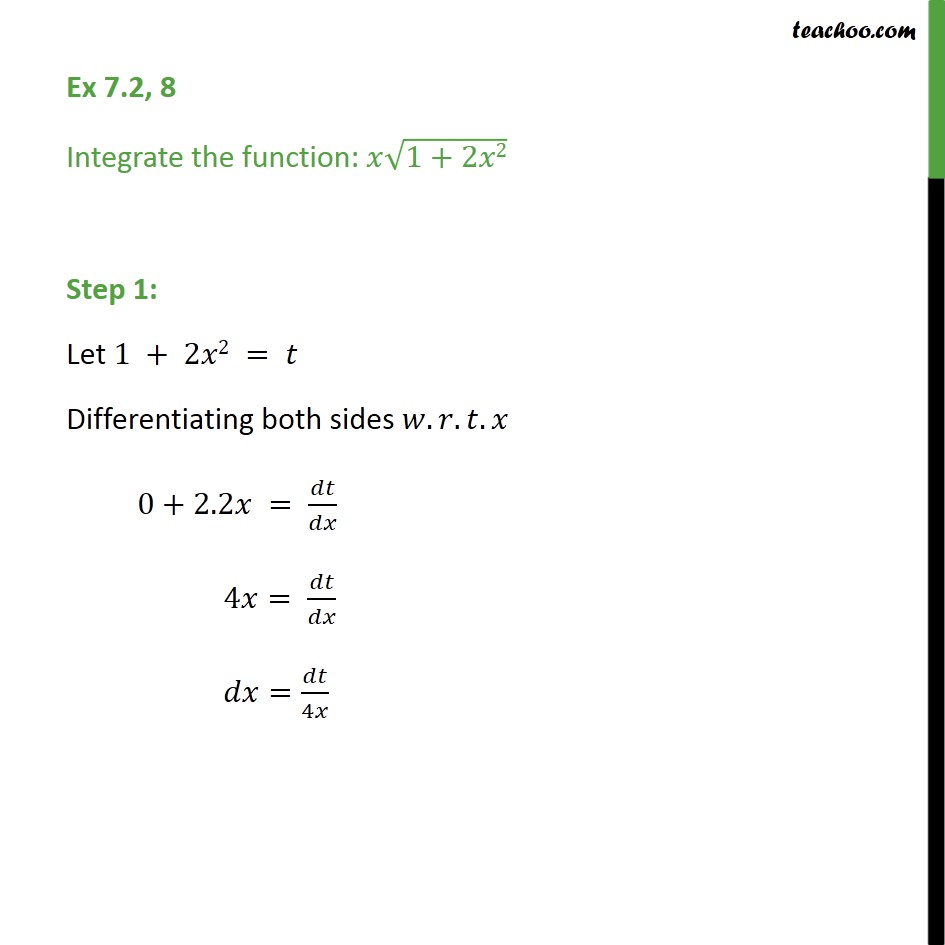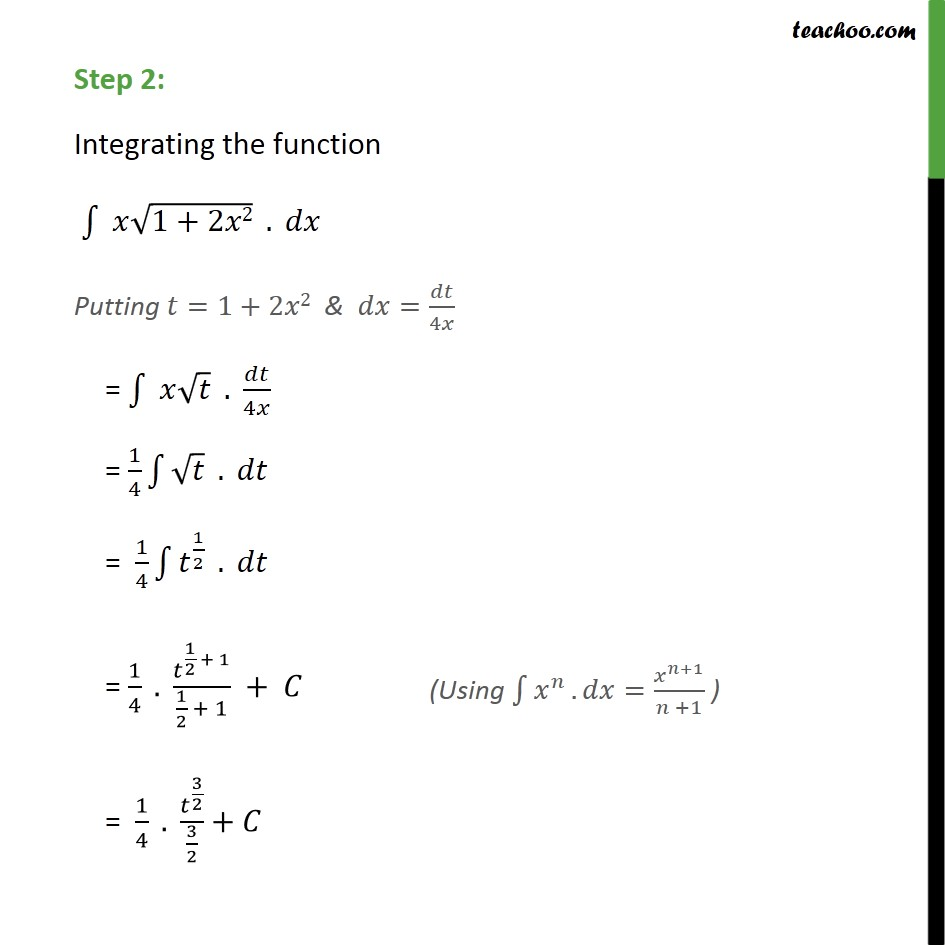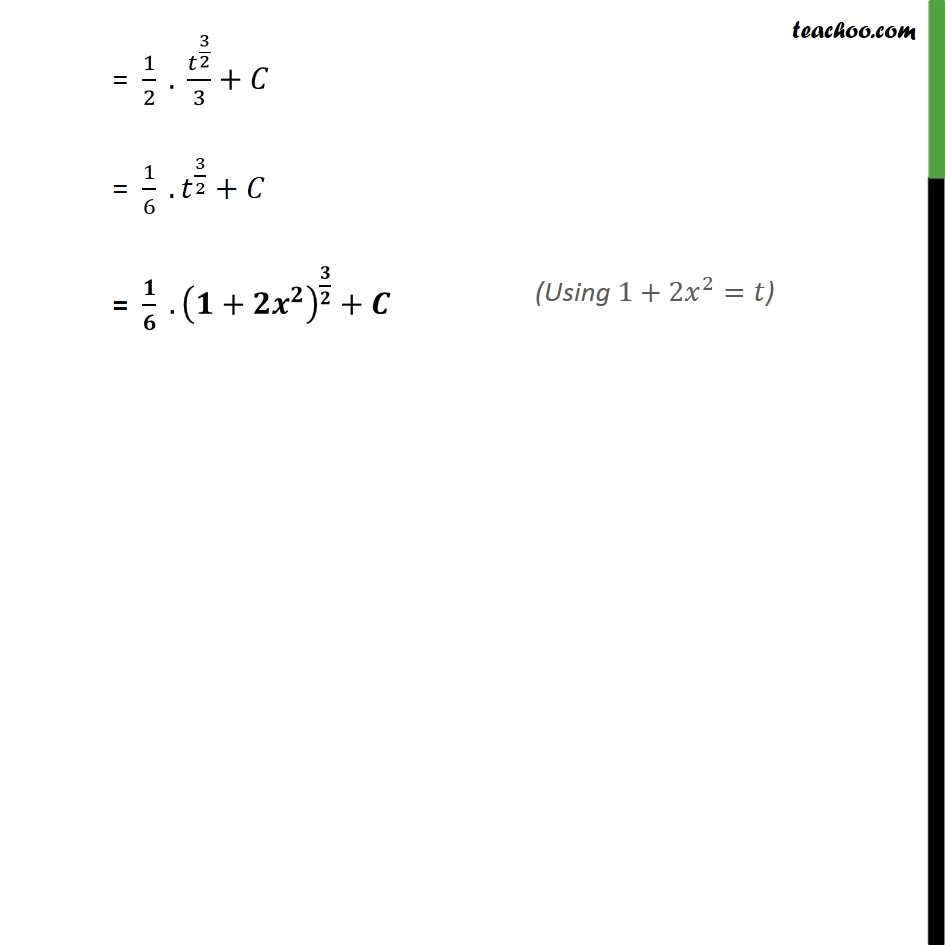Integration Full Chapter Explained - Integration Class 12 - Everything you need1. Chapter 7 Class 12 Integrals
2. Serial order wise
3. Ex 7.2

Transcript

Ex 7.2, 8 Integrate the function: 1+2 2 Step 1: Let 1 + 2 2 = Differentiating both sides . . . 0+2.2 = 4 = = 4 Step 2: Integrating the function 1+2 2 . Putting =1+2 2 & = 4 = . 4 = 1 4 . = 1 4 1 2 . = 1 4 . 1 2 + 1 1 2 + 1 + = 1 4 . 3 2 3 2 + = 1 2 . 3 2 3 + = 1 6 . 3 2 + = . + +

Ex 7.2

Chapter 7 Class 12 Integrals
Serial order wise